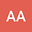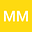CMMSE: Estimates of singular numbers ( s -numbers) and eigenvalues of a mixed elliptic-hyperbolic type operator with parabolic degeneration
•••## Abstract

a) | k ( w ) | ≥ 0 is a piecewise continuous and bounded function in R = ( - ∞ , ∞ ) . The coefficients b ( w ) and q ( w ) are continuous functions in R and can be unbounded at infinity. The operator L admits closure in the space L 2 ( Ω ) and the closure is also denoted by L. Taking into consideration certain constraints on the coefficients b ( w ) q ( w ) , apart from the above-mentioned conditions, the existence of a bounded inverse operator is proved in this paper; a condition guaranteeing compactness of the resolvent kernel is found; and we also obtained two-sided estimates for singular numbers ( s-numbers). Here we note that the estimate of singular numbers ( s-numbers) shows the rate of approximation of the resolvent of the operator L by linear finite-dimensional operators. It is given an example of how the obtained estimates for the s-numbers enable to identify the estimates for the eigenvalues of the operator L. We note that the above results are apparently obtained for the first time for a mixed-type operator in the case of an unbounded domain with rapidly oscillating and greatly growing coefficients at infinity.
10 Sep 2022Submitted to Mathematical Methods in the Applied Sciences
10 Sep 2022Submission Checks Completed
10 Sep 2022Assigned to Editor
21 Sep 2022Reviewer(s) Assigned
15 Oct 2022Review(s) Completed, Editorial Evaluation Pending
16 Oct 2022Editorial Decision: Revise Minor